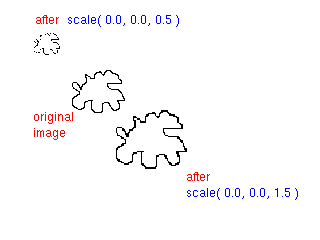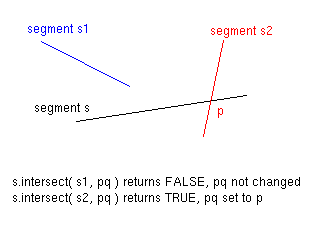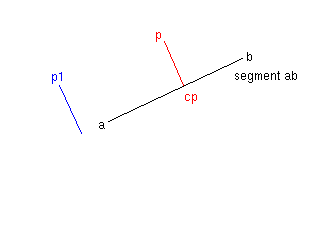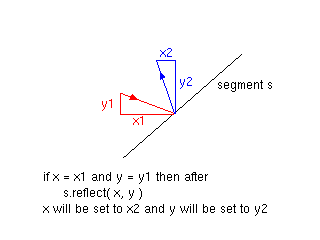## 7. Shapes

### 7.1. Limitations

The shape classes form a polymorphic collection to display graphical shapes on a graphics window. They are used for many examples and exercises in this book.

These classes are definitely not a model for a professional graphics library. In particular, the Point class (and, to a lesser degree, the Segment and Rectangle classes) serve double duty: both for the actual shapes to be plotted and for geometric computation. For the latter role, the polymorphism overhead is entirely unnecessary. For example, a polygon is stored as an array of Point objects, each of which has a pointer to an unneeded virtual function table. It would make more sense to distinguish between a Point (an object representing a point in the plane, with geometric operations such as distance and angle measurement), and a PointShape (a blob on the graphics screen). For simplicity, ChiLib does not make this distinction.

The class pairs Rectangle/FilledRect and Text/ScalableText had been introduced in the book as examples for inheritance. In practice, fixed-size text is nearly useless, and it would make sense to make all text scalable. If a distinction between rectangles and rectangle shapes were made, one might also decide to make all rectangle shapes filled.

For geometric calculations, and as the argument to the move operation, it would have been very convenient to have a Vector class, with overloaded operators for vector addition, scaling and dot product. But the distinction between points and vectors is subtle. For example, it makes no sense to add two points, whereas the difference between two points is defined--it is a vector. It was reluctantly decided that introducing vectors would obscure those issues that this library intends to illustrate.

The classes and class operations have further been restricted by the rudimentary capabilities of the least capable of the graphics library that ChiLib supports. That library does not offer rotated text; hence we do not implement a rotate operation on shapes, although that would be an interesting and natural operation to carry out. If it were added, the implementation of ellipses and rectangles would be affected. Professional graphics libraries support arbitrary affine transformations of the plane.

Mathematical purity demands geometric operations to be independent from a particular coordinate system. Our interface succeeds, with two exceptions. The Point default constructor constructs a point at the origin, and the rectangles, the ellipses and the move operation depend on the direction of the x- and y-axes.

### 7.2. Input and output

```      void read(istream& is);
void plot(GraphicsContext& gc) const;
void print(ostream& os) const;
```

These three operations must be defined for every class deriving from Shape. The most important output routine is plotting on a graphics context. Use the graphics context operations to render the shape. If you modify the state of the graphics context, restore it to the default.

To print on a stream, write the object state in a form that is both humanly readable (for easier debugging) and easy to read back in. The read operation restores an object from the information in a file in the format produced by print. The old contents of the object are lost. If the contents of the input file do not match the expected format, the stream state should be set to `fail'.

### 7.3. Transformations

```
void scale(Point center, double s);
void move(double x, double y);
```

In addition to being plottable, shapes can be transformed. We support two transformations only: moving and scaling. Moving is the easiest. The shape is transported by a given x- and y-offset. In figure 3, we represent the shape as an amorphous blob to indicate that any shape can be transformed in this way.Figure 3. The move operation

The scale operation has two ingredients: the center of projection and a scale factor. All points of the shape are moved further away from the center, if the scale factor is > 1, or closer to the center, if the scale factor is < 1. (Only positive scale factors are allowed.) Note that the center can be any point and need not be the origin of the coordinate system.Figure 4. The scale operation

Each class that derives from Shape must supply its own move and scale operations. Moving is usually easy--just move the points that define the shape. To scale, scale the points, and multiply all length measurements (such as the radius of a circle) by the scale factor.

For most shapes, the only operations that can change them after construction are move and scale. (The exception is the polygon, whose vertices can be set individually.)

### 7.4. Default constructors

All shape classes have default constructors, but they all construct objects that are not useful. The Point constructor constructs a point on the origin. Since the interface is independent of the choice of an origin, this is not likely ever to be useful. If you mean to place a point at (0, 0), construct it with explicit arguments.

The Segment default constructor makes an empty segment joining two copies of the origin. The default Ellipse is centered on the origin, with radii 0. Neither shape is useful for anything.

We supplied the default constructors for two reasons. Some applications may need arrays of certain shapes; for example, a maze may be an array of segments. Second, it is necessary to construct an object first before invoking the read operation.

### 7.5. Cloning

Every class deriving from Shape should redefine the virtual clone function as follows:

```
class MyShape : public Shape
{
public:
// ...
MyShape* clone() const;
};

MyShape* clone() const { return new MyShape(self); }
```

(Older compilers may require that you declare the return value as Shape*, not MyShape*. If the compiler demands that, make the modification. It does not affect our applications.)

Cloning is needed for the graph editor framework and for any application in which a deep copy of a shape is required.

The Shape base class defines clone to return 0. As long as you are not interested in cloning, you need not redefine it. In particular, it is not an issue for the first eight chapters.

### 7.6. Points

```
Point(double xx, double yy);
double x() const;
double y() const;
double distance(Point b) const;
double angle(Point b) const;
```

The x and y accessors report the coordinates of the point.

The distance operation measures the distance between to another point. The angle operation computes the angle between the x-axis and the segment joining this point with the point b, counted clockwise. (This is the opposite of the mathematical convention because the screen y-axis points downward.) The angle is measured in radians.

### 7.7. Segments

```
Segment(Point f, Point t);
Point from() const;
Point to() const;
Point center() const;
Bool intersect(Segment s, Point& p) const;
Point closest(Point p) const;
double distance(Point p) const;
void reflect(double& x, double& y) const;
```

A segment is an interval on a line joining two points. The from and to accessors return the end points, and center returns the point halfway between.

The intersect operation computes the intersection between two segments. It returns TRUE, and sets p to the point of intersection, if the segments intersect. Otherwise it returns FALSE and leaves p unchanged.Figure 5. Intersection of segments

The closest operation computes the point on the segment that is closest to p.Figure 6. Closest point on a segment

This is the point c such that the segment cp is perpendicular to the segment ab, provided c lies between a and b. Otherwise the operation returns the closer of the end points a, b.

The distance operation computes the distance between p and the closest point to p on the segment. If the distance is (approximately) 0, then p lies on the segment.

The reflect operation reflects a vector, given by its x- and y-coordinates, along the segment.Figure 7. Reflection along a segment

The x and y arguments are changed to the reflected vector. This operation is useful for bouncing shapes off a boundary.

### 7.8. Rectangles

```
Rectangle(Point, Point);
Point left_top() const;
Point left_bottom() const;
Point right_top() const;
Point right_bottom() const;
Point center() const;
double xsize() const;
double ysize() const;
Bool is_inside(Point p) const;
Point boundary_point(Point p) const;
```

All rectangles have their sides parallel to the coordinate axes and can thus be defined by any two corner points (not necessarily top left and bottom right). The four corner points are returned as left_top, left_bottom, right_top, right_bottom. (The left/right comes first because it depends on the x value, and top/bottom depends on the y value.)

The center operation returns the center of the rectangle.

The xsize, xysize operations return the lengths of the sides of the rectangle.

The is_inside operation checks whether p is inside the rectangle. The boundary_point operation returns the point on the boundary of the rectangle intersecting the segment joining p and the center of the rectangle. It returns p if p is inside the rectangle.

### 7.9. Filled rectangles

```
FilledRect(Point, Point, GraphicsContext::BrushStyle);
```
Filled rectangles are just like rectangles, except that the fill pattern must be specified in the constructor.

### 7.10. Ellipses

```
Point center() const;
Bool is_inside(Point p) const;
Point boundary_point(Point p) const;
```

All ellipses have their axes parallel to the coordinate axes. To construct an ellipse, specify the center and the radii. If the radii are equal, a circle is obtained.

The center operation returns the center of the ellipse, The xradius and yradius operations return the radii of the ellipse.

The is_inside operation checks whether p is inside the ellipse. The boundary_point operation returns the point on the boundary of the ellipse intersecting the segment joining p and the center of the ellipse. The operation returns p if p is inside the ellipse.

### 7.11. Polygons

```
Polygon(int);
void set_vertex(int, Point);
Point vertex(int i) const;
Point center() const;
```

To construct a polygon, specify the number of vertices that is desired. (Admittedly, this constructor violates the rule that a constructor should not have a single integer argument.) Then call set_vertex to set each vertex.

The vertex operation returns the ith vertex. center computes the center of gravity.

### 7.12. Text

```
Text(Point, String);
Rectangle extent(GraphicsContext& gc) const;
```

Text is a string placed on a specific location on the screen. To construct a text object, specify both the top left corner and the contents.

The extent operation returns the rectangle enclosing the text. You must supply a graphics context to enable measurement.

### 7.13. Scalable text

```
ScalableText(Point, String, double yheight = 1.0);
```
The characters of Text shapes are formed with the default system font. Their size is not affected by scaling. The ScalableText characters overcome that problem. You can specify the character height in the constructor, and it is correctly updated when the shape is scaled. For example, character height 1 means that the character box (including ascenders and descenders) is one unit high.

The plot and extent operations of the Text class are redefined to work with scalable text.